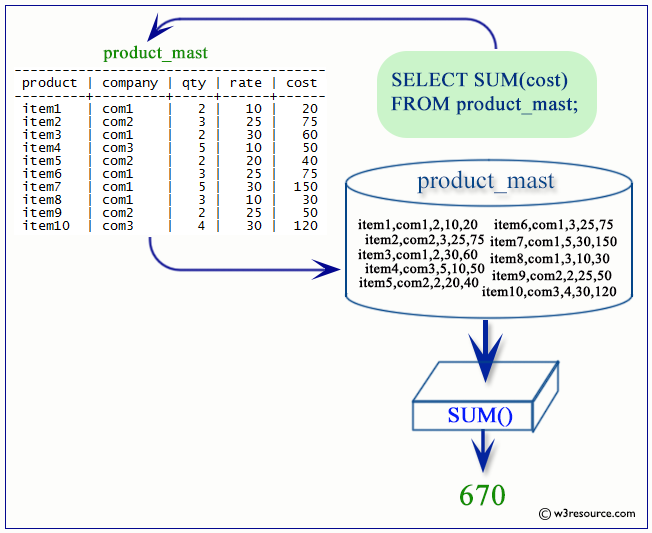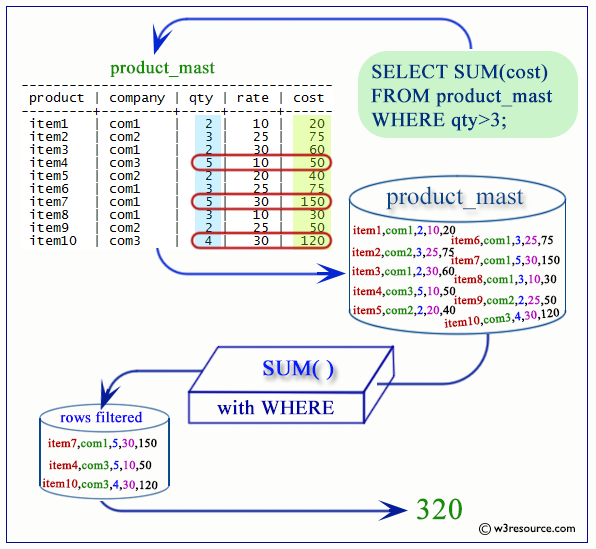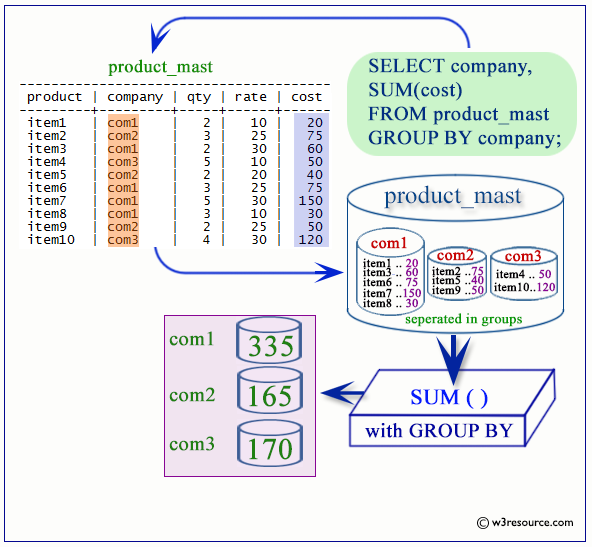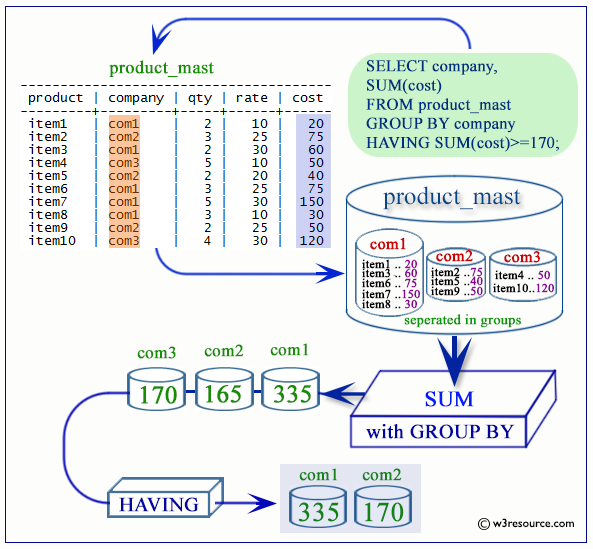# PostgreSQL SUM function

## SUM function

The SUM function in PostgreSQL returns the sum of values for the list of selected columns.

Syntax

```SUM (* | [DISTINCT] ALL | column_name)
```

Parameters

Name Description
column_name Name of the column
* The asterisk(*) indicates all the rows.
DISTINCT This clause is optional. It indicates uniqueness.
ALL This clause is optional. It is default clause.

PostgreSQL SUM function example

The sample tableIf we want to calculate the total sum of salary for all employees in the employee table, the following SQL can be used.

SQL

Code:

``````SELECT SUM(salary)
FROM employee;
```
```

Output:Pictorial Presentation of PostgreSQL SUM()PostgreSQL SUM as a level

If we want to calculate the total sum of salary for all employees and show the result against 'Total Salary' head in the employee table, the following SQL can be used.

SQL

Code:

``````SELECT SUM(salary)  AS "Total Salary"
FROM employee;
```
```

Output:PostgreSQL SUM DISTINCT

If we want to get the sum of unique salary from employee table, the following SQL can be used.

SQL

Code:

``````SELECT SUM ( DISTINCT salary)
FROM employee;
```
```

Output:Explanation

The above example shows that the sum of salary is smaller than the sum salary for all employees because the DISTINCT clause eliminates the repetition of each salary and summed only once.

PostgreSQL SUM WHERE clause

If we want to get the sum of unique salary from employee table who belongs to the deptno 15, the following SQL can be used.

SQL

Code:

``````SELECT SUM ( DISTINCT salary) AS "Total Salary"
FROM employee
WHERE deptno=15;
```
```

Output:Pictorial Presentation of PostgreSQL SUM with WHEREPostgreSQL SUM with GROUP BY

Sample table: employees

The following statement will return the designation and total salary against each designation from the employees table.

SQL

Code:

``````SELECT job_id,SUM(salary) AS "Total Salary"
FROM employees
GROUP BY job_id;;
```
```

Sample Output:

```   job_id   | Total Salary
------------+--------------
AC_ACCOUNT |      8300.00
ST_MAN     |     36400.00
IT_PROG    |     28800.00
SA_MAN     |     61000.00
AC_MGR     |     12000.00
FI_MGR     |     12000.00
MK_MAN     |     13000.00
PU_CLERK   |     13900.00
HR_REP     |      6500.00
PR_REP     |     10000.00
FI_ACCOUNT |     39600.00
SH_CLERK   |     64300.00
SA_REP     |    250500.00
ST_CLERK   |     55700.00
MK_REP     |      6000.00
PU_MAN     |     11000.00
(19 rows)
```

Pictorial Presentation of PostgreSQL SUM with GROUP BYPostgreSQL SUM with WHERE CLAUSE and GROUP BY

Sample table: employees

The following statement will return the designation wise total salary for that designation which salary does not exceed 12000 and above, from employees table.

SQL

Code:

``````SELECT job_id,SUM(salary) AS "Total Salary"
FROM employees
WHERE salary<12000
GROUP BY job_id;
```
```

Sample Output:

```   job_id   | Total Salary
------------+--------------
AC_ACCOUNT |      8300.00
ST_MAN     |     36400.00
IT_PROG    |     28800.00
SA_MAN     |     21500.00
PU_CLERK   |     13900.00
HR_REP     |      6500.00
PR_REP     |     10000.00
FI_ACCOUNT |     39600.00
SH_CLERK   |     64300.00
SA_REP     |    250500.00
ST_CLERK   |     55700.00
MK_REP     |      6000.00
PU_MAN     |     11000.00
(14 rows)
```

PostgreSQL SUM with HAVING CLAUSE and GROUP BY

Sample table: employees

The following SQL statement will return, designation wise total employees and total salary for that designation which salary does not exceed 12000 and above and a minimum of five employees have this designation.

SQL

Code:

``````SELECT job_id,COUNT(*) AS "Number of Employees",
SUM(salary) AS "Total Salary"
FROM employees
WHERE salary<12000
GROUP BY job_id
HAVING COUNT(*)>=5;
```
```

Sample Output:

```   job_id   | Number of Employees | Total Salary
------------+---------------------+--------------
ST_MAN     |                   5 |     36400.00
IT_PROG    |                   5 |     28800.00
PU_CLERK   |                   5 |     13900.00
FI_ACCOUNT |                   5 |     39600.00
SH_CLERK   |                  20 |     64300.00
SA_REP     |                  30 |    250500.00
ST_CLERK   |                  20 |     55700.00
(7 rows)
```

Pictorial Presentation of PostgreSQL SUM with HAVINGPostgreSQL SUM with GROUP BY and ORDER BY

Sample table: employees

The following statement will return, the designation wise total employees and total salary, arranged in descending order according to the total number of employees, for that designation which salary does not exceed 12000 and above and a minimum of five employees have this designation.

SQL

Code:

``````SELECT job_id,COUNT(*) AS "Number of employees" ,
SUM(salary) AS "Total Salary"
FROM employees
WHERE salary<12000
GROUP BY job_id
HAVING COUNT(*)>=5
ORDER BY COUNT(*) DESC;
```
```

Sample Output:

```   job_id   | Number of employees | Total Salary
------------+---------------------+--------------
SA_REP     |                  30 |    250500.00
SH_CLERK   |                  20 |     64300.00
ST_CLERK   |                  20 |     55700.00
ST_MAN     |                   5 |     36400.00
FI_ACCOUNT |                   5 |     39600.00
IT_PROG    |                   5 |     28800.00
PU_CLERK   |                   5 |     13900.00
(7 rows)
```

Previous: COUNT
Next: MAX

﻿